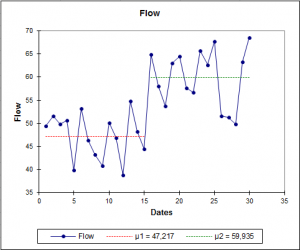# Homogeneity tests for time series

Homogeneity tests for time series test allow to detect a change along a time series. Available in Excel using the XLSTAT statistical software.## What are homogeneity tests for time series

Homogeneity tests enables you to determine if a series may be considered as homogeneous over time, or if there is a time at which a change occurs.

Homogeneity tests involve a large number of tests, XLSTAT offer four tests (Pettitt, Buishand, SNHT, or von Neumann), for which the null hypothesis is that a time series is homogenous between two given times.

The variety of the tests comes from the fact that there are many possible alternative hypotheses: changes in distribution, changes in average (one or more times) or presence of trend.

## Homogeneity tests p-value approximation

The tests presented in this tool correspond to the alternative hypothesis of a single shift. For all tests, XLSTAT provides p-values using Monte Carlo resamplings. Exact calculations are either impossible or too costly in computing time.

Note 1: If you have a clear idea of the time when the shift occurs, one can use the tests available in the parametric or nonparametric tests sections. For example, assuming that the variables follow normal distributions, one can use the test z (known variance) or the Student t test (estimated variance) to test the presence of a change at time t. If one believes that the variance changes, you can use a comparison test of variances (F-test in the normal case, for example, or Kolmogorov-Smirnov in a more general case).

Note 2: The tests presented below are sensitive to a trend (for example a linear trend). Before applying these tests, you need to be sure you want to identify a time at which there is a shift between two homogeneous series.

## Pettitt’s test

The Pettitt's test is a nonparametric test that requires no assumption about the distribution of data. The Pettitt's test is an adaptation of the tank-based Mann-Whitney test that allows identifying the time at which the shift occurs. In his article of 1979 Pettitt describes the null hypothesis as being that the T variables follow the same distribution F, and the alternative hypothesis as being that at a time t there is a change of distribution. Nevertheless, the Pettitt test does not detect a change in distribution if there is no change of location. For example, if before the time t, the variables follow a normal N(0,1) distribution and from time t a N (0,3) distribution, the Pettitt test will not detect a change in the same way a Mann-Whitney would not detect a change of position in such a case. In this case, one should use a Kolmogorov Smirnov based test or another method able to detect a change in another characteristic than the location. We thus reformulate the null and alternative hypotheses:

H0: The T variables follow one or more distributions that have the same location parameter.

Two-tailed test: Ha: There exists a time t from which the variables change of location parameter.

Left-tailed test: Ha: There exists a time t from which the variables location is reduced by D.

Left-tailed test: Ha: There exists a time t from which the variables location is augmented by D.

The SNHT test (Standard Normal Homogeneity Test) was developed by Alexandersson (1986) to detect a change in a series of rainfall data. The test is applied to a series of ratios that compare the observations of a measuring station with the average of several stations. The ratios are then standardized. The series of Xi corresponds here to the standardized ratios. The null and alternative hypotheses are determined by:

H0: The T variables Xi follow a N(0,1) distribution.

Ha: Between times 1 and n the variables follow an N(µ1, 1) distribution, and between n+1 and T they follow an N(µ2,1) distribution.

## Buishand’s test

The Buishand’s test (1982) can be used on variables following any type of distribution. But its properties have been particularly studied for the normal case. In his article, Buishand focuses on the case of the two-tailed test, but for the Q statistic presented below the one-sided cases are also possible. Buishand has developed a second statistic R, for which only a bilateral hypothesis is possible.

In the case of the Q statistic, the null and alternative hypotheses are given by:

H0: The T variables follow one or more distributions that have the same mean. Two-tailed test: Ha: There exists a time t from which the variables change of mean.

Left-tailed test: Ha: There exists a time t from which the variables mean is reduced by D.

Left-tailed test: Ha: There exists a time t from which the variables mean is augmented by D.

## von Neumann’s ratio test

The von Neumann ratio test is very powerful at all times but does not allow detecting the time of the change.### analyze your data with xlstat

14-day free trial

Included in

Related features